o
osc_x4h57ch8

## 一、复习

``````b = 1
c = 2
d = 3
a = b+c
print(a)
e = a + d
print(e)``````

3
6

``````import logging
logging.basicConfig(level=logging.DEBUG,filename = 'userinfo.log')
b = 1
c = 2
d = 3
a = b+c
logging.debug(a)
e = a + d
logging.warning(e)``````

DEBUG:root:3
WARNING:root:6

hashlib 摘要 md5 sha系列
文件的一致性校验
密文的认证
logging 记录日志
两种用法 basicConfig不常用 getLogger()常用
可以通过一个参数去控制全局的日志输出情况
可以帮助开发者同时向文件和屏幕输出信息
configparser
有分组section和有配置项option的配置文件，默认.ini结尾的文件
collections
在基础数据类型之外又额外增加了一些新的数据类型

``````from collections import OrderedDict
d = OrderedDict()
d['a'] = 1
d['z'] = 2
d['a'] = 3
print(d)``````

OrderedDict([('a', 3), ('z', 2)])

``````from collections import OrderedDict
d = OrderedDict()
d['a'] = 1
d['z'] = 2
d['a'] = 3
d['z'] = 0  # 修改值
print(d)``````

OrderedDict([('a', 3), ('z', 0)])

## 二、defaultdict

kuriko有如下值集合 [11,22,33,44,55,66,77,88,99,90...]，将所有大于 66 的值保存至字典的第一个key中，将小于 66 的值保存至第二个key的值中。

``````dic = {}
li = [11,22,33,44,55,66,77,88,99,90]
for i in li:
if i > 66:
if dic.get('k1'):
dic['k1'].append(i)
else:
dic['k1'] = [i]
else:
if dic.get('k2'):
dic['k2'].append(i)
else:
dic['k2'] = [i]
print(dic)``````

{'k1': [77, 88, 99, 90], 'k2': [11, 22, 33, 44, 55, 66]}

``````from collections import defaultdict
li = [11,22,33,44,55,66,77,88,99,90]
d = defaultdict(list)  # callable() 接收一个可调用对象。给每一个即将要用key，设置一个默认值
for i in li:
if i > 66:
d['k1'].append(i)
else:
d['k2'].append(i)
print(d)``````

defaultdict(<class 'list'>, {'k2': [11, 22, 33, 44, 55, 66], 'k1': [77, 88, 99, 90]})

``````from collections import defaultdict
li = [11,22,33,44,55,66,77,88,99,90]
d = defaultdict(set)
for i in li:
if i > 66:
else:
print(d)``````

defaultdict(<class 'set'>, {'k1': {88, 90, 99, 77}, 'k2': {33, 66, 11, 44, 22, 55}})

``````from collections import defaultdict
d = defaultdict(set)
print(d)``````

defaultdict(<class 'set'>, {})

``````from collections import defaultdict
d = defaultdict(set)
print(d['a'])
d['b'] = 10
print(d)``````

set()
defaultdict(<class 'set'>, {'a': set(), 'b': 10})

``````from collections import defaultdict
defaultdict([])
dic = {}
l = []
dic = {'k1':1,'k2':1}``````

TypeError: first argument must be callable or None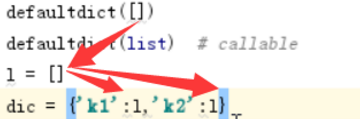``````from collections import defaultdict
d = defaultdict(list)
print(d)
print(d)  # 值不存在
print(d)``````

defaultdict(<class 'list'>, {})
[]
defaultdict(<class 'list'>, {1: []})

defaultdict 只能接收可调用的对象，由于lambda 也是可调用的

``````from collections import defaultdict
d = defaultdict(lambda : 5)
print(d)``````

## 三、Counter

Counter类的目的是用来跟踪值出现的次数。它是一个无序的容器类型，以字典的键值对形式存储，其中元素作为key，其计数作为value。计数值可以是任意的Interger（包括0和负数）。Counter类和其他语言的bags或multisets很相似。

``````from collections import Counter
c = Counter('ab')
print(c)``````

Counter({'a': 1, 'b': 1})

``````from collections import Counter
c = Counter('which')
c.update('witch') # 使用另一个iterable对象更新
print(c['h'])  # 统计h出现了几次``````

``````from collections import Counter
c = Counter('which')
d = Counter('watch')
c.update(d) # 使用另一个Counter对象更新
print(c['h'])  # 统计h出现了几次``````

## 四、时间模块

``````import time#常用方法
1.time.sleep(secs)
(线程)推迟指定的时间运行。单位为秒。
2.time.time()

给人看的   格式化的时间

给机器看的  时间戳最接近计算机的时间

计算用户 元组 最接近 python

(1)时间戳(timestamp) ：通常来说，时间戳表示的是从1970年1月1日00:00:00开始按秒计算的偏移量。我们运行“type(time.time())”，返回的是float类型。
(2)格式化的时间字符串(Format String)： ‘1999-12-06’``````%y 两位数的年份表示（00-99）
%Y 四位数的年份表示（000-9999）
%m 月份（01-12）
%d 月内中的一天（0-31）
%H 24小时制小时数（0-23）
%I 12小时制小时数（01-12）
%M 分钟数（00=59）
%S 秒（00-59）
%a 本地简化星期名称
%A 本地完整星期名称
%b 本地简化的月份名称
%B 本地完整的月份名称
%c 本地相应的日期表示和时间表示
%j 年内的一天（001-366）
%p 本地A.M.或P.M.的等价符
%U 一年中的星期数（00-53）星期天为星期的开始
%w 星期（0-6），星期天为星期的开始
%W 一年中的星期数（00-53）星期一为星期的开始
%x 本地相应的日期表示
%X 本地相应的时间表示
%Z 当前时区的名称
%% %号本身``````
python中时间日期格式化符号：

1970年1月1 是英国时间，
(3)元组(struct_time) ：struct_time元组共有9个元素共九个元素:(年，月，日，时，分，秒，一年中第几周，一年中第几天等）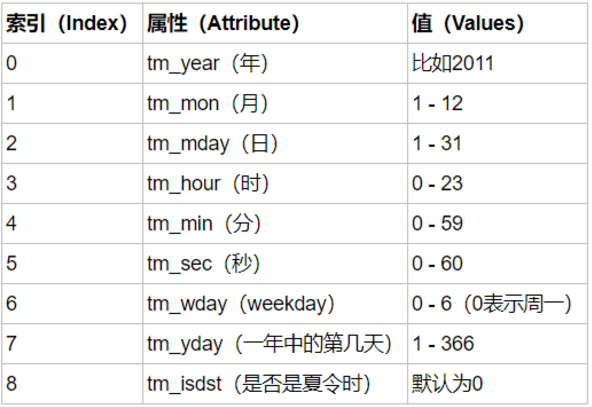几种格式之间的转换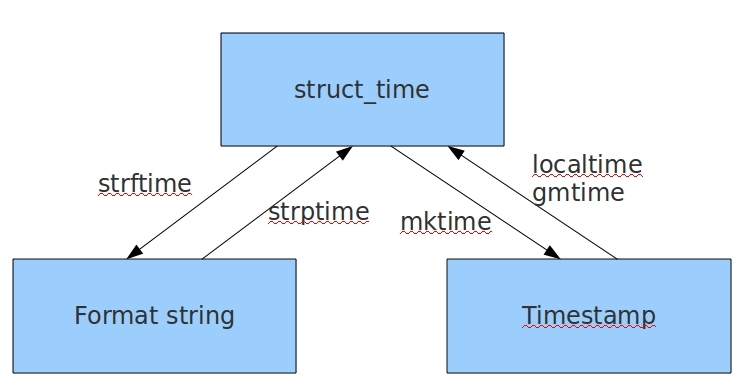``````import time
print(time.time())  # 时间戳时间
print(time.strftime('%Y-%m-%d'))  # 字符串格式化时间
print(time.strftime('%m/%d/%Y'))  # 月/天/年
print(time.strftime('%H:%M:%S'))  # 时:分:秒
print(time.strftime('%Y-%m-%d %H:%M:%S'))  # 标准时间
print(time.strftime('%I:%M:%S'))  # 十二小时制
print(time.strftime('%x'))  # 本地相应的日期表示
print(time.strftime('%X'))  # 本地相应的时间表示
print(time.strftime('%x %X'))  # 本地日期和时间
print(time.strftime('%c'))  # 本地相应的日期表示和时间表示
print(time.localtime())  # 结构化时间``````

1524563384.8112636
2018-04-24
04/24/2018
17:49:44
2018-04-24 17:49:44
05:49:44
04/24/18
17:49:44
04/24/18 17:49:44
Tue Apr 24 17:49:44 2018
time.struct_time(tm_year=2018, tm_mon=4, tm_mday=24, tm_hour=17, tm_min=49, tm_sec=44, tm_wday=1, tm_yday=114, tm_isdst=0)

``(2018,4,24,10,39,46,1,114,0)``

%Y %m %d %H %M %S

``````import time
print(time.gmtime())  # 英国的结构化时间
print(time.localtime())  # 本地结构化时间,时间戳时间转格式化时间的中间件``````

``````import time
p = time.strptime('2015-8-8','%Y-%m-%d')  # 时间字符串->结构化时间
print(p)
print(time.mktime(p))  # 结构化时间->时间戳

print(time.time() - time.mktime(p))  # 当前时间戳-上面的时间戳``````

time.struct_time(tm_year=2015, tm_mon=8, tm_mday=8, tm_hour=0, tm_min=0, tm_sec=0, tm_wday=5, tm_yday=220, tm_isdst=-1)
1438963200.0
85605561.47843862

``````ret = time.localtime(1500000000)  # 时间戳->结构化时间
print(ret)
print(time.strftime('%Y-%m-%d %H:%M:%S',ret))  # 结构化时间->时间字符串``````

time.struct_time(tm_year=2017, tm_mon=7, tm_mday=14, tm_hour=10, tm_min=40, tm_sec=0, tm_wday=4, tm_yday=195, tm_isdst=0)
2017-07-14 10:40:00

``````ret = time.localtime(1600000000)
print(ret)
print(time.strftime('%Y-%m-%d %H:%M:%S',ret))``````

time.struct_time(tm_year=2020, tm_mon=9, tm_mday=13, tm_hour=20, tm_min=26, tm_sec=40, tm_wday=6, tm_yday=257, tm_isdst=0)
2020-09-13 20:26:40

``````ret = time.localtime(0)
print(ret)
print(time.strftime('%Y-%m-%d %H:%M:%S',ret))``````

time.struct_time(tm_year=1970, tm_mon=1, tm_mday=1, tm_hour=8, tm_min=0, tm_sec=0, tm_wday=3, tm_yday=1, tm_isdst=0)
1970-01-01 08:00:00

0就表示1970年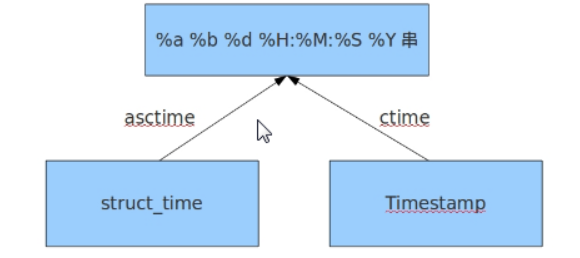time.asctime(结构化时间) 如果不传参数，直接返回当前时间的格式化串

``````import timeprint(time.strftime('%c'))
print(time.ctime())
print(time.asctime())``````

Tue Apr 24 19:29:29 2018
Tue Apr 24 19:29:29 2018
Tue Apr 24 19:29:29 2018

time.ctime(时间戳)  如果不传参数，直接返回当前时间的格式化串

``````print(time.ctime(1500000000))
ret = time.localtime(2000000000)
print(ret)
print(time.asctime())
print(time.asctime(ret))``````

Fri Jul 14 10:40:00 2017
time.struct_time(tm_year=2033, tm_mon=5, tm_mday=18, tm_hour=11, tm_min=33, tm_sec=20, tm_wday=2, tm_yday=138, tm_isdst=0)
Tue Apr 24 19:31:37 2018
Wed May 18 11:33:20 2033

## 五、random模块

random() 方法返回随机生成的一个实数，默认它在[0,1)范围内。

打印顺序，比如洗牌

随机，比如彩票，验证码

``````import random
#随机小数
print(random.random())  # 大于0且小于1之间的小数
print(random.uniform(1,3))  #大于1小于3的小数,可以用来发红包``````

0.7056803343481585
1.4427911142943668

``````#随机整数
print(random.randint(1,5))  # 大于等于1且小于等于5之间的整数
print(random.randrange(1,10,2))  # 大于等于1且小于10之间的奇数``````

4
3

``````print(random.randint(1,5))  # 大于等于1且小于等于5之间的整数
print(random.randrange(1,10,2)) # 大于等于1且小于10之间的奇数``````

5
1

``````print(random.choice([1,'23',[4,5]]))  # #1或者23或者[4,5]
print(random.sample([1,'23',[4,5]],2))  # #列表元素任意2个组合``````

[4, 5]
['23', [4, 5]]

sample，可以做抽奖。比如公司年终奖，三等奖10名，直接抽取10个就可以了。

``````#打乱列表顺序
item=[1,3,5,7,9]
random.shuffle(item) # 打乱次序
print(item)
print(random.shuffle(item))
print(item)``````

[9, 3, 7, 5, 1]
None
[1, 9, 5, 7, 3]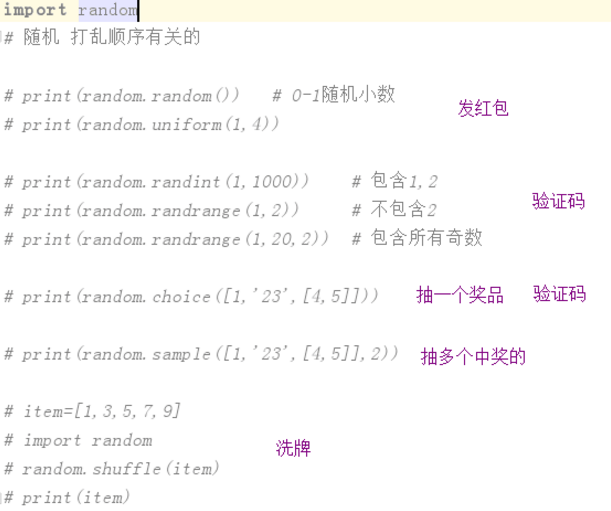## 六、sys模块

sys模块是与python解释器交互的一个接口

很多人会把os模块和sys模块弄混，不知道该用哪一个？

os模块  操作系统打交道的

sys模块 python解释器打交道的

sys.argv 命令行参数List，第一个元素是程序本身路径
sys.exit(n) 退出程序，正常退出时exit(0),错误退出sys.exit(1)
sys.version 获取Python解释程序的版本信息
sys.path 返回模块的搜索路径，初始化时使用PYTHONPATH环境变量的值
sys.platform 返回操作系统平台名称

``````import sys
print('*'*6)
sys.exit()  # 下面的代码不会执行
print('-'*6)``````

******

``````import sys
print(sys.version)``````

3.5.4 (v3.5.4:3f56838, Aug 8 2017, 02:17:05) [MSC v.1900 64 bit (AMD64)]

``````import sys
print(sys.platform)``````

win32

win32表示是Windows系统，linux2表示是linux平台

sys.path

``````import sys
print(sys.path)``````

['E:\\python_script\\day26', 'E:\\python_script', 'C:\\Python35\\python35.zip', 'C:\\Python35\\DLLs', 'C:\\Python35\\lib', 'C:\\Python35', 'C:\\Python35\\lib\\site-packages', 'C:\\Program Files\\JetBrains\\PyCharm 2018.1.1\\helpers\\pycharm_matplotlib_backend']

sys.path 把系统的路径加进去了，所以import的时候，能搜索到

sys.argv

sys.argv当前程序名
sys.argv第一个参数
sys.argv第二个参数
...

test.py内容如下：

``````import sys
print(sys.argv)``````

python test.py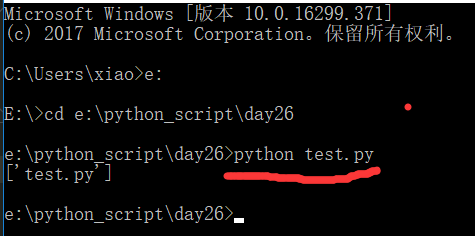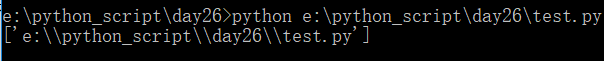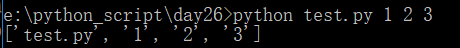比如登录程序，可以通过传参的方式，验证。

test.py文件内容如下：

``````import sys
print(sys.argv)   # 列表 列表的第一项是当前文件所在的路径
if sys.argv == 'alex' and sys.argv == '3714':
print('登陆成功')
else:
sys.exit()
user = input('>>>')
pwd = input('>>>')
if user == 'alex' and pwd == '3714':
print('登陆成功')
else:
sys.exit()
print('我能完成的功能')``````

python test.py alex 3714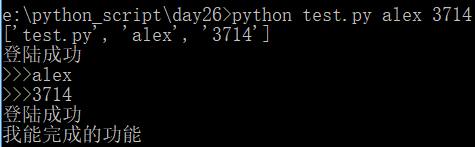典型用法：

debug

直接执行

test.py代码如下：

``````import logging
num = int(input('>>>'))
a = num * 100
b = a - 10
c = b + 5
print(c)``````

``````import sys
import logging
inp = sys.argv.upper() if len(sys.argv)>1 else 'WARNING' # 日志级别
logging.basicConfig(level=getattr(logging,inp))  # DEBUG
num = int(input('>>>'))
logging.debug(num)
a = num * 100
logging.debug(a)
b = a - 10
logging.debug(b)
c = b + 5
print(c)``````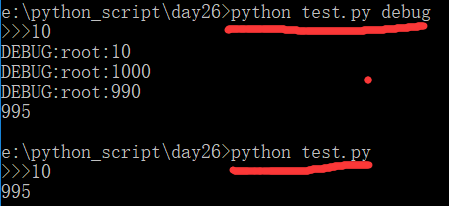``````import sys
import logging

lev = sys.argv.upper() if len(sys.argv) > 1 else 'WARNING'
logging.basicConfig(level=getattr(logging, lev))
num = int(input('>>>').strip())
logging.debug(num)
a = num * 100
logging.debug('{}*100={}'.format(num, a))
b = a - 10
logging.debug('{}-10={}'.format(a, b))
c = b + 5
logging.debug('{}+5={}'.format(b, c))
print(c)``````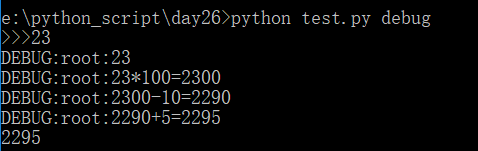sys模块中,重点要掌握的就是argv和path

``````1.要求 生成随机验证码

2.y-m-d h:M:S 比如2017-07-08 10:23:41

1.1 数字，主要有0~9组成，使用random.randint生成

``print(random.randint(0,9))``

1.2 使用random模块取6个

``````import random
random_list = []
for i in range(6):  # 取6次
random_num = random.randint(0,9)  #  大于等于0且小于等于9之间的整数
random_list.append(random_num)  # 追加到列表

print(random_list)``````

[5, 9, 5, 0, 5, 4]

1.3 将列表转换为字符串，使用join。注意，使用join，必须保证列表中的每一个元素为字符串类型

``````import random
random_list = []
for i in range(6):  # 取6次
random_num = random.randint(0,9)  #  大于等于0且小于等于9之间的整数
random_list.append(str(random_num))  # 将元素转换为str并追加到列表

ret = ''.join(random_list)
print(ret)``````

615411

1.4进阶需求，如何输出所有的字母呢？需要用到ascci码

``````capital = [chr(i) for i in range(65,91)]  #所有大写字母
lowercase = [chr(i) for i in range(97,123)]  #所有小写字母

print(capital)
print(lowercase)``````

['A', 'B', 'C', 'D', 'E', 'F', 'G', 'H', 'I', 'J', 'K', 'L', 'M', 'N', 'O', 'P', 'Q', 'R', 'S', 'T', 'U', 'V', 'W', 'X', 'Y', 'Z']
['a', 'b', 'c', 'd', 'e', 'f', 'g', 'h', 'i', 'j', 'k', 'l', 'm', 'n', 'o', 'p', 'q', 'r', 's', 't', 'u', 'v', 'w', 'x', 'y', 'z']

1.6 将数字和字母组合，生成4位

``````import random
for i in range(4):  # 取4位
lowercase = chr(random.randint(97,123))  #所有小写字母
capital = chr(random.randint(65,91))  #所有大写字母
number = str(random.randint(0,9))  # 所有数字
print(lowercase,capital,number)``````

w J 8
z O 5
e T 6
u S 9

1.7 将随机数添加到列表中，并转化为字符串

``````import random
code = []
for i in range(4):  # 取4位
lowercase = chr(random.randint(97,123))  #所有小写字母
capital = chr(random.randint(65,91))  #所有大写字母
number = str(random.randint(0,9))  # 所有数字
code.append(random.choice([lowercase,capital,number]))  # 有3个元素，几率为1/3
ret = ''.join(code)  # 列表转换为字符串
print(ret)``````

7JmY

2.1既然是比较2个时间的差值，就需要转化为时间戳，再做减法

``````import time
before = time.strptime("2017-07-08 10:23:41",'%Y-%m-%d %H:%M:%S')
before_stamp = time.mktime(before)
print(before_stamp)``````

1499480621.0

2.2 将before_stamp 和当前时间戳相减

``````import time
before = time.strptime("2017-07-08 10:23:41",'%Y-%m-%d %H:%M:%S')
before_stamp = time.mktime(before)

diff_stamp = time.time() - before_stamp # 当前时间戳-指定时间戳
print(diff_stamp)``````

25095583.435019016

2.3 差值转换为英国的结构化时间

``````import time
before = time.strptime("2017-07-08 10:23:41",'%Y-%m-%d %H:%M:%S')
before_stamp = time.mktime(before)

diff_stamp = time.time() - before_stamp # 当前时间戳-指定时间戳
struct_time=time.gmtime(diff_stamp)  # 差值转换为英国的结构化时间
print(struct_time)``````

time.struct_time(tm_year=1970, tm_mon=10, tm_mday=18, tm_hour=11, tm_min=2, tm_sec=53, tm_wday=6, tm_yday=291, tm_isdst=0)

2.4 时间戳为0时，表示1970-01-01 0:00:00

``````import time
before = time.strptime("2017-07-08 10:23:41",'%Y-%m-%d %H:%M:%S') # 之前的时间
before_stamp = time.mktime(before)  # 转换为时间戳

diff_stamp = time.time() - before_stamp # 当前时间戳-指定时间戳
struct_time=time.gmtime(diff_stamp)  # 差值转换为英国的结构化时间

#1970-01-01 0:00:00  # 英国时间
year = struct_time.tm_year - 1970
month = struct_time.tm_mon - 1
day = struct_time.tm_mday - 1
hour = struct_time.tm_hour
minute = struct_time.tm_min
second = struct_time.tm_sec

print('过去了{}年{}月{}日{}时{}分{}秒'.format(year,month,day,hour,minute,second))``````

o

### osc_x4h57ch8#### 暂无文章

Hacker News 简讯 2020-07-11

FalconChen

142
0

29
0
【LeetCode】53 盛最多水的容器

20
0

16
0
js 整数与小数正则替换片段

DrChenXX

17
0